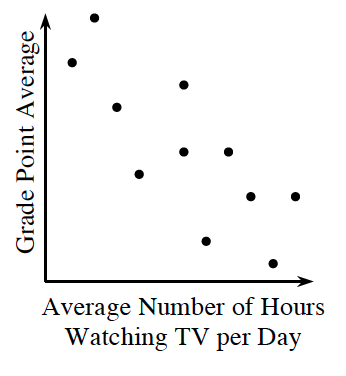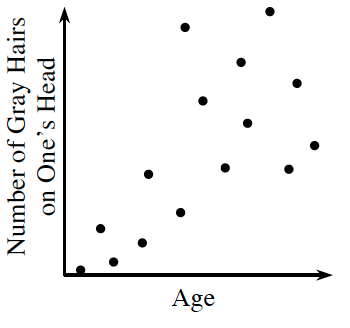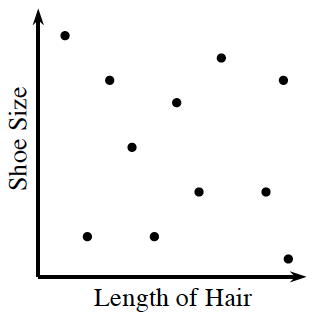### Home > CC3 > Chapter Ch7 > Lesson 7.1.3 > Problem7-29

7-29.

For each scatterplot below, determine if there is an association between the points. Label each graph as showing a positive association, negative association, or no association. If there is an association, write a sentence describing it.

1.As the number of hours watching TV increases, the grade point average decreases.
The graph has a negative correlation.

1.Positive correlation.

1.Is there any trend in the graph above?